### Non-Harmonic Periodic Forcing Functions: Fourier Analysis

To this point we have only considered harmonic forcing functions (e.g. sine). These are examples of periodic functions since they repeat themselves after a specific period of time. However, harmonic functions are not the only type of periodic function. There are many others, some typical examples of which are shown in Figure 6.1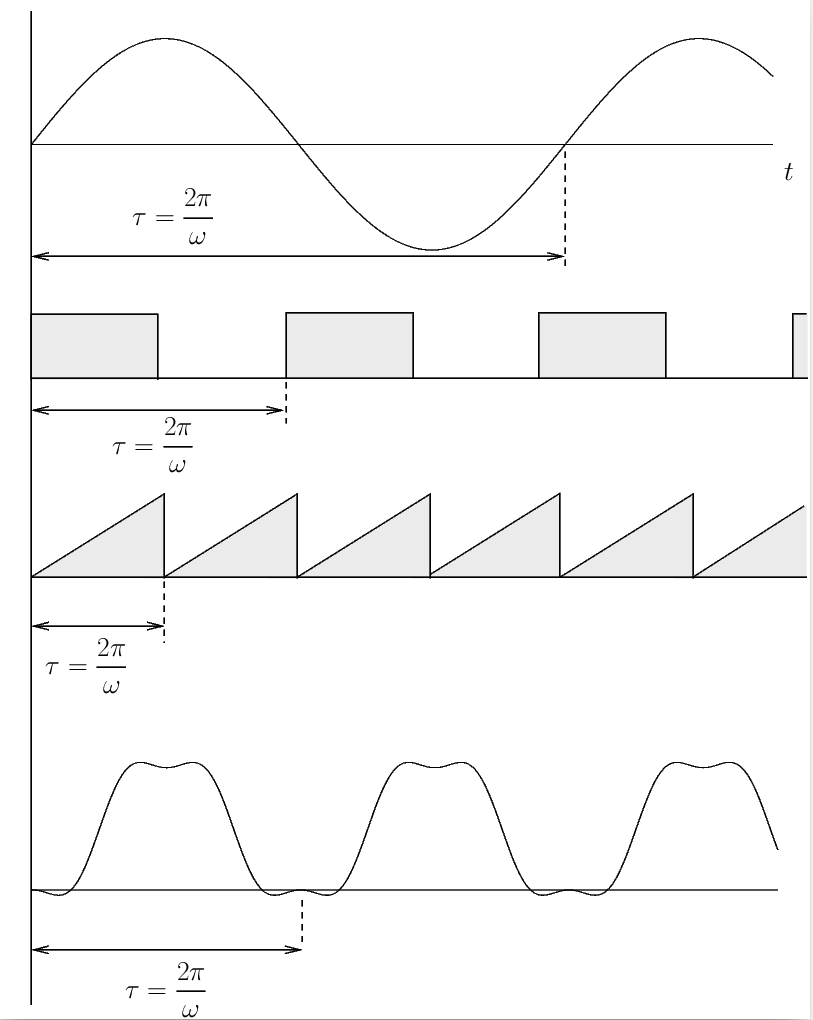Figure 6.1: Examples of periodic functions. From top to bottom: harmonic function, square wave, sawtooth, and a general periodic function.

It would be useful to be able to determine the response of a single degree of freedom system to these other periodic forcing functions as well. Fortunately much of what we have already studied can be applied.

### Fourier Analysis

Whenever we have a periodic forcing function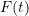with period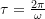it can always be expressed as a Fourier series (a sum of harmonic functions) as

(6.1)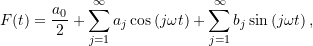where

(6.2a)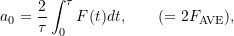(6.2b)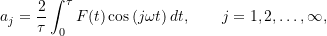(6.2c)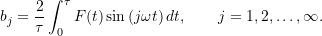For a given periodic function, the coefficients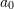,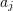and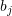(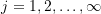) can be uniquely determined.

With this expansion of the forcing function, the equation of motion for the damped spring-mass system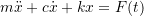becomes

(6.3)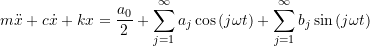To find the steady state solution to (6.3) we make use of the principle of superposition to express the total solution as the sum of the individual solutions to each term on the right hand side. That is, the solution is the sum of the solutions to

(6.4a)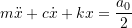(6.4b)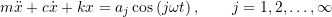(6.4c)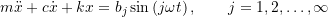The steady state solution to (6.4a) is simply

(6.5)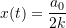Aside
We have previously shown that the steady state solution to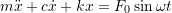is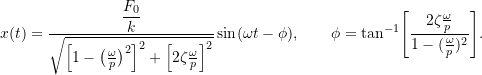Following the same procedure, it is straightforward to show that the solution to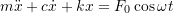is similarly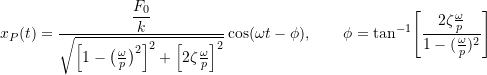The steady state solution to equations (6.4b) and (6.4c) are

(6.6)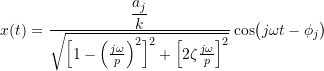and

(6.7)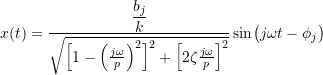respectively where

(6.8)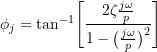Therefore the steady state solution to equation (6.3) is given by

(6.9)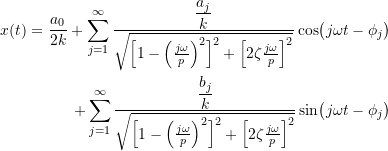where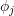is given by (6.8)

Note:

• The amplitude and phase angle for the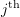frequency depend on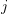and are not constants
• The terms with frequency component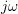closest to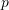will have the largest dynamic magnification factor.
• Ascontinues to increase, the amplitude of theterms decreases (due to the dynamic magnification factor). As a result, in practice a reasonably accurate solution can be obtained using only the first few terms in the expansion in equation (6.3).
• If the periodic forcing function is sufficiently complex or is determined experimentally the integrations in equations (6.2b) and (6.2c) may not be available analytically. In these case the Fourier coefficients can be approximated numerically.

We can consider the periodto be broken into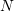(an even number) of equally spaced time intervals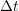so that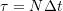.

If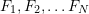represent the values ofat the times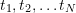then the Fourier coefficients can be approximated as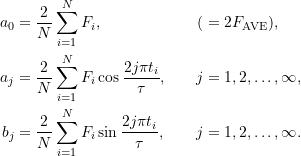Once these coefficients are known, we can proceed as before withreplaced by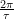.
• The solutions shown are for the steady state only. If we are interested in the transient solution as well, we must add the solution to

(6.10)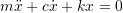to our solution. The solution to (6.10) for the underdamped case is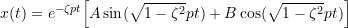in which the two constants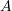and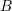are determined using initial conditions. These constants must be determined using the complete solution, so the Fourier coefficients need to be determined beforeandcan be evaluated.

#### EXAMPLE

A 3.68 kg slider is placed in a smooth tube with an internal diameter of 40 mm. The spring has a stiffness of 284 N/m. One side of the tube is open to the atmosphere. The pressure on the other side varies periodically as shown in the graph.

Determine the steady state response of the slider